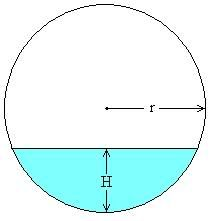# Thread: Calculate depth of water in pipe if pipe radius and X-sectional area of water known?

1. ##Calculate depth of water in pipe if pipe radius and X-sectional area of water known?

Does anyone have a direct solution for this problem?

Consider a pipe of radius r, parially filled with water. The cross-sectional area of the water standing in the pipe is known. What is depth H of the water standing in the pipe?I've been able to solve for H using an iterative method, but I have not found a direct solution formula. Does anyone have a formula to calculate H is r and the area of the shaded blue area are known?

Thanks,
John

2.Originally Posted by JohnM77Does anyone have a direct solution for this problem?

Consider a pipe of radius r, parially filled with water. The cross-sectional area of the water standing in the pipe is known. What is depth H of the water standing in the pipe?I've been able to solve for H using an iterative method, but I have not found a direct solution formula. Does anyone have a formula to calculate H is r and the area of the shaded blue area are known?

Thanks,
John
Hi JohnM77,

If you draw the radii from the circle centre to the left and right edges of the surface of the water in your diagram, draw the radius from the centre to the bottom of the pipe, you have 2 back-to-back right-angled triangles sitting on the water with top apex at the pipe centre.

Label the angle at the centre for either triangle A.
Then you work it through as the triangle heights are r-H.

The cross-sectional area of the water equals the (sector area)-(the sum of the triangle areas)

$\displaystyle V_w=\frac{1}{2}r^2(2A)-r(r-H)SinA=r^2A-r(r-H)SinA$

$\displaystyle \Rightarrow\ r(r-H)SinA=r^2A-V_w$

$\displaystyle \Rightarrow\ \frac{r^2A-V_w}{rSinA}=r-H$

$\displaystyle H=r-\frac{r^2A-V_w}{rSinA}$

where $\displaystyle A=Cos^{-1}\left(\frac{r-H}{H}\right)$

3. Thanks very much for your reply. I follow the logic of your method, but it with angle A being a function of H, is this still not a direct solution?

4. Yes, John,
you're quite right,
this needs completion.

,

,

,

,

,

,

,

,

,

# finding depth of water in a pipe

Click on a term to search for related topics.

#### Search Tags

area, calculate, depth, pipe, radius, water, xsectional